# E-PolyLearning

 1. Heat flow across a hollow sphere of inner radius 'r1' and outer radius 'r2' is directly proportional to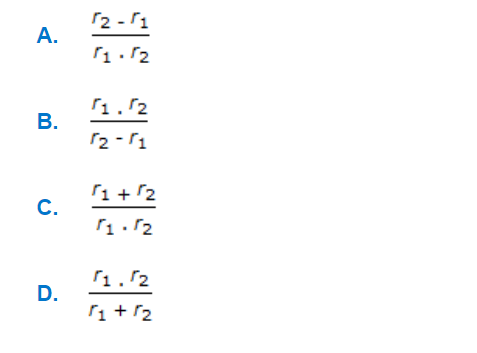a. A b. B c. C d. D

 2. Work done by a/an __________ process is determined by following equation.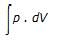a. adiabatic b. quasi-static c. isothermal d. isentropic
 3. Which of the following is the correct nature of shear stress distribution along the cross section in a horizontal circular pipe under steady state fluid flow condition ?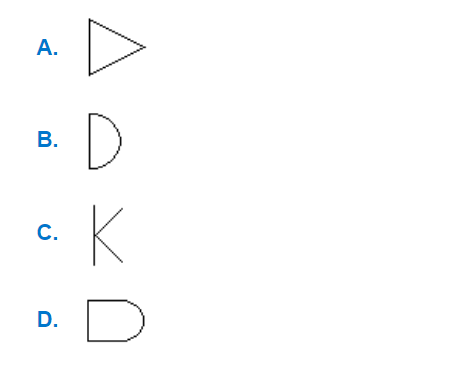a. A b. B c. C d. D
 4. The unit of gc is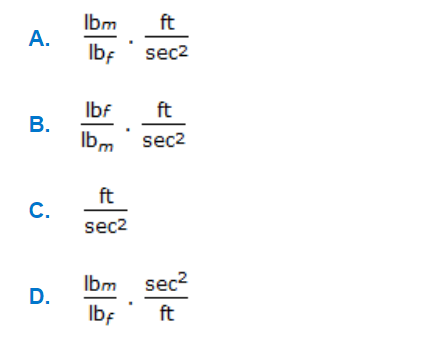a. A b. B c. C d. D
 5. Temperature profile along the length of a gas-gas counter flow heat exchanger is correctly represented by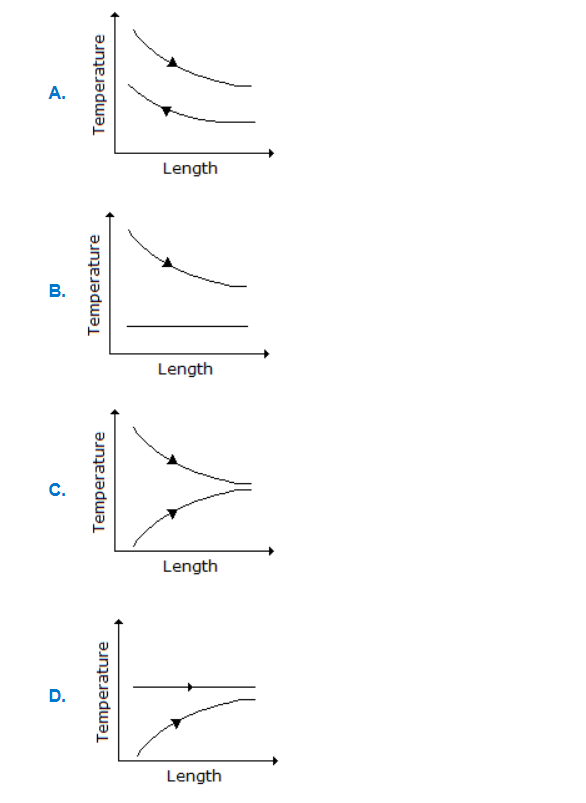a. A b. B c. C d. D